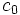# Orbital maximax is bounded below by constant fraction of number of ordered pairs of distinct elements for groups of fixed prime power order

## Statement

Suppose$p$ is a (fixed) prime number. Consider the Orbital maximax problem (?) for finite$p$-groups acting on a set$S$ of size$n$: we want to find the maximum possible size of the largest orbital under the action of a finite$p$-group on$S$.

The claim is that there is a constant$c_0$ (depending on$p$, and in fact of the order of$p^2$), such that for any$n$, there is a group action such that size of the largest orbital is$\ge n(n-1)/c_0$.

## Related facts

### Related facts about orbital maximax

Type of group Result
abelian group Orbital maximax equals size of set for abelian groups